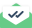# compute heat/flux command

Dear Lammps users

I am, trying to calculate thermal conductivity using Green-Kubo method using compute heat/flux command. There is an input script given at the end of compute heat/flux command description. Can anybody please clarify why we have used f_JJ, f_JJ,f_JJ in vaiables k11, k22, and k33. The input script is pasted below.

Thank you.

Truly,
Rajesh.

# Sample LAMMPS input script for thermal conductivity of solid Ar

units real
variable T equal 70
variable V equal vol
variable dt equal 4.0
variable p equal 200 # correlation length
variable s equal 10 # sample interval
variable d equal \$p*\$s # dump interval

# convert from LAMMPS real units to SI

variable kB equal 1.3806504e-23 # [J/K] Boltzmann
variable kCal2J equal 4186.0/6.02214e23
variable A2m equal 1.0e-10
variable fs2s equal 1.0e-15
variable convert equal {kCal2J}*{kCal2J}/{fs2s}/{A2m}

# setup problem

dimension 3
boundary p p p
lattice fcc 5.376 orient x 1 0 0 orient y 0 1 0 orient z 0 0 1
region box block 0 4 0 4 0 4
create_box 1 box
create_atoms 1 box
mass 1 39.948
pair_style lj/cut 13.0
pair_coeff * * 0.2381 3.405
timestep \${dt}
thermo \$d

# equilibration and thermalization

velocity all create \$T 102486 mom yes rot yes dist gaussian
fix NVT all nvt temp \$T \$T 10 drag 0.2
run 8000

# thermal conductivity calculation, switch to NVE if desired

#unfix NVT
#fix NVE all nve

reset_timestep 0
compute myKE all ke/atom
compute myPE all pe/atom
compute myStress all stress/atom NULL virial
compute flux all heat/flux myKE myPE myStress
variable Jx equal c_flux/vol
variable Jy equal c_flux/vol
variable Jz equal c_flux/vol
fix JJ all ave/correlate \$s \$p d & c_flux c_flux c_flux type auto file J0Jt.dat ave running variable scale equal {convert}/\${kB}/\$T/\$T/\$V*s*{dt}
variable k11 equal trap(f_JJ){scale} variable k22 equal trap(f_JJ)*{scale}
variable k33 equal trap(f_JJ)
\${scale}
thermo_style custom step temp v_Jx v_Jy v_Jz v_k11 v_k22 v_k33
run 100000
variable k equal (v_k11+v_k22+v_k33)/3.0
variable ndens equal count(all)/vol
print “average conductivity: \$k[W/mK] @ T K, {ndens} /A^3”

Fix JJ is an instance of fix ave/correlate. The
fix ave/correlate doc page explains its output (see
the bottom of the page). Part of the text says:

The global array has # of rows = Nrepeat and # of columns = Npair+2. The first column has the time delta (in timesteps) between the pairs of input values used to calculate the correlation, as described above. The 2nd column has the number of samples contributing to the correlation average, as described above. The remaining Npair columns are for I,J pairs of the N input values, as determined by the type keyword, as described above.

So the output you want starts in the 3rd column.

Steve

Dear Steve,
Thank you for clarification.

One more point I noticed is that in doc page description in the kappa formula volume term is in numerator while in the script given below it is in denominator. I think doc page formula has some error as in literature also volume term will be found in denominator. Please correct me if I am wrong.

Thanks
Rajesh

The doc page formula for kappa = V * (J dot J)

and J has a 1/V, so kappa = V / V^2 = 1/V ?

Steve

Dear Steve

Thank you for clarification. I got it. V factor in not included in heat/flux compute and thats why it has been divided twice by vol in lammps script. One more thing I have a question. Why do calculated Jx, Jy and Jz and average them even if we need to calculate kappa for a single direction only??

Thank you.

Rajesh

If you are modeling an isotropic system, ideally, the kappa values along x,y,z should be equal. Therefore, to reduce the uncertainty of the results of MD simulations, we could average the three kappas. However, if your system is anisotropic, this averaging would be a wrong practice.

It is worth mentioning that comparing the kappa values could provide a good means for evaluating the precision of the results of our simulations.

Regards

MNSent with Mailtrack

Dear Mostafa,

Thank you for your suggestion. Actually, my kappa values are different in all the three directions with large difference. So, it will be better for me to use direction wise kappa values.

One more query is regarding convergence. Of which quantity, the convergence should be attained?? J or kappa??

Thank you.

Rajesh.

Dear Rajesh

your welcome. Actually, you will have a different value for J at any time step while the entire simulation gives only one kappa (in any direction). Therefore, it is the convergence of kappa which you should be concern about. For a better perception of this topic you could read the following article (especially look at Fig. 7):

http://journals.aps.org/prb/abstract/10.1103/PhysRevB.65.144306

Also, in chapter 7 of the following book you could find very useful materials:

Haile, J. M. "Molecular dynamics simulation: elementary methods. 1992

Regards

MNSent with Mailtrack